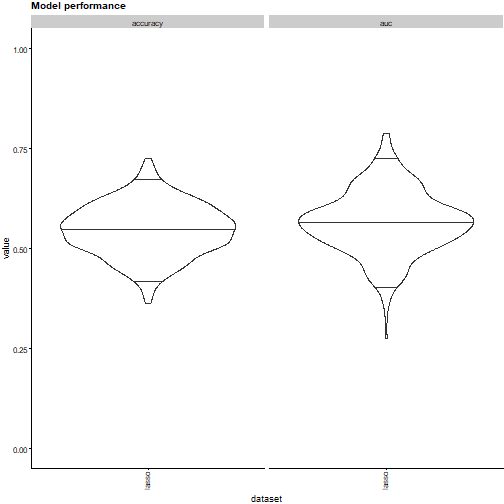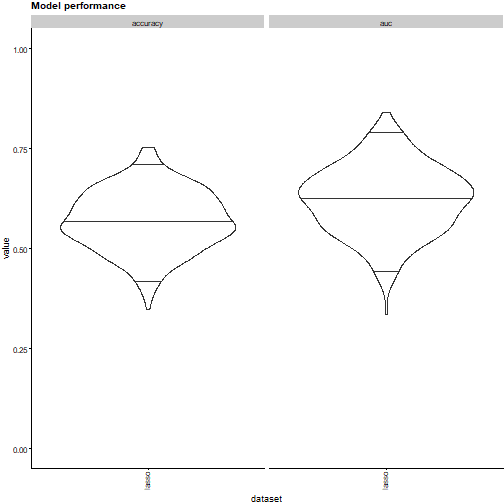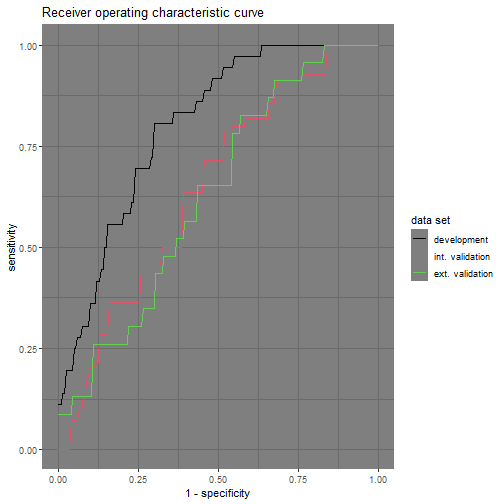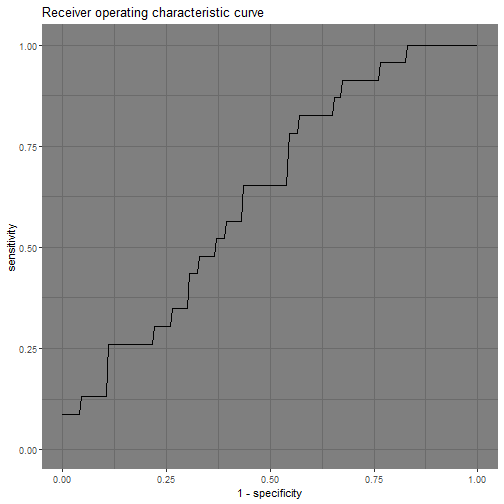# Using familiar prospectively

#### 2022-04-07

library(familiar)
library(data.table)

set.seed(19)

The summon_familiar function is used to generate models for a given dataset and provide a broad analysis afterwards. This creates a number of files. Many of the files resulting from this analysis can also be used outside of summon_familiar. For example, models and ensembles can be used prospectively to assess new datasets. Likewise, data and collection objects can be used to customise plotting and export to tables.

In this example we will use the birthweight dataset collected in 1986 at Baystate Medical Center, Springfield, Massachusetts. This dataset contains birth weight data from 189 newborn children, some of which have low birth weight (less then 2.5 kg). Potential risk factors were also collected. We will try to predict the low birth weight indicator using these risk factors.

We will first randomly split the data into development and validation datasets:

# Load the birth weight data set
data <- data.table::as.data.table(MASS::birthwt)

# Add sample and batch identifiers.
data[,":="("sample_id"=.I)]

# Generate training and validation samples
train_samples <- sample(data$sample_id, size=120, replace=FALSE) valid_samples <- setdiff(data$sample_id, train_samples)

# Assign batch identifiers.
data[sample_id %in% train_samples, "batch_id":="development"]
data[sample_id %in% valid_samples, "batch_id":="validation"]

Next we will prepare the dataset further. We drop the bwt column as this directly contains the indicator we are trying to predict. Other columns are encoded as categorical variables.

# Drop the bwt column.
data[, "bwt":=NULL]

# Encode the low outcome column: we ensure that "low" is now the so-called
# positive class.
data$low <- factor(data$low, levels=c(0, 1), labels=c("normal", "low"))

# Encode race. smoke, ht, and ui columns as categorical variables.
data$race <- factor(data$race, levels=c(1, 2, 3), labels=c("white", "black", "other"))
data$smoke <- factor(data$smoke, levels=c(0, 1), labels=c("no", "yes"))
data$ht <- factor(data$ht, levels=c(0, 1), labels=c("no", "yes"))
data$ui <- factor(data$ui, levels=c(0, 1), labels=c("no", "yes"))

# Rename columns to make them clearer.
data.table::setnames(data,
old=c("low", "age", "lwt", "race", "smoke", "ptl", "ht", "ui", "ftv"),
new=c("birth_weight", "age_mother", "weight_mother_before_pregnancy",
"ethnicity", "smoking_during_pregnancy", "previous_premature_labours",
"hypertension_history", "uterine_irritability", "physician_visits_first_trimester"))

Then we call summon_familiar create models for the data and assess these. We will create an ensemble of five penalised logistic regression models to predict the low birth weight indicator, based on bootstraps of the development dataset. You may notice that we here write to the temporary R directory using the tempdir() function. In practice you will want to use a different directory, as the temporary R directory will be deleted once your R session closes. For speed, we will also only compute point estimates during evaluation (see the evaluation and explanation vignette for other options).

familiar::summon_familiar(data=data,
project_dir=tempdir(),
sample_id_column="sample_id",
batch_id_column="batch_id",
development_batch_id="development",
outcome_type="binomial",
outcome_column="birth_weight",
experimental_design="bs(fs+mb,5) + ev",
cluster_method="none",
fs_method="none",
learner="lasso",
parallel=FALSE,
estimation_type="point")

# Using models prospectively

Models generated by familiar are stored in subdirectories of the trained_models folder:

# Create path to the directory containing the models.
model_directory_path <- file.path(tempdir(), "trained_models", "lasso", "none")

# List files present in the directory.
list.files(model_directory_path)
#>   "20220407171238_hyperparameters_lasso_none_2_1.RDS" "20220407171238_hyperparameters_lasso_none_2_2.RDS" "20220407171238_hyperparameters_lasso_none_2_3.RDS" "20220407171238_hyperparameters_lasso_none_2_4.RDS"
#>   "20220407171238_hyperparameters_lasso_none_2_5.RDS" "20220407171238_lasso_none_1_1_ensemble.RDS"        "20220407171238_lasso_none_2_1_model.RDS"           "20220407171238_lasso_none_2_2_model.RDS"
#>   "20220407171238_lasso_none_2_3_model.RDS"           "20220407171238_lasso_none_2_4_model.RDS"           "20220407171238_lasso_none_2_5_model.RDS"

There are 5 models in the directory, which are stored in RDS format in files ending with *_model.RDS. We can inspect the first model in the directory.

# Create path to the model.
model_path <- file.path(model_directory_path, list.files(model_directory_path, pattern="model"))

model
#> A lasso model (class: familiarGLMnetLasso; v1.1.0) trained using glmnet (v4.1.3) package.
#>
#> --------------- Model details ---------------
#>
#>
#> ---------------------------------------------
#>
#> The following outcome was modelled:
#> birth_weight (binomial), with classes: normal (reference) and low.
#>
#> The model was trained using the following hyperparameters:
#>   sign_size: 8
#>   family: binomial
#>   lambda_min: lambda.min
#>   n_folds: 7
#>   normalise: FALSE
#>   sample_weighting: inverse_number_of_samples
#>   sample_weighting_beta: -2
#>
#> Variable importance was determined using the none variable importance method.
#>
#> The following features were used in the model:
#> previous_premature_labours (numeric).
#> ethnicity (categorical), with levels: white (reference), black and other.
#> age_mother (numeric):
#>   transformation (yeo_johnson) with <U+03BB> = -0.8.
#>   normalisation (standardisation) with shift = 1.15040432726622 and scale = 0.0170214246457444.
#> smoking_during_pregnancy (categorical), with levels: no (reference) and yes.
#> hypertension_history (categorical), with levels: no (reference) and yes.
#> physician_visits_first_trimester (numeric):
#>   transformation (yeo_johnson) with <U+03BB> = -0.8.
#>   normalisation (standardisation) with shift = 0.313192082386248 and scale = 0.324948104478325.
#> uterine_irritability (categorical), with levels: no (reference) and yes.
#> weight_mother_before_pregnancy (numeric):
#>   transformation (yeo_johnson) with <U+03BB> = -1.2.
#>   normalisation (standardisation) with shift = 0.830774795606794 and scale = 0.00055659494321713.
#>
#> A novelty detector was trained using the model features.

This model can then be used to predict values for a given dataset, among other things. The predict method used by familiar is in many ways similar to other predict methods. However, familiar requires that the newdata argument is set. It does not store development data with its models to limit model size and prevent leaking sensitive information. Predictions can be made as follows:

predict(object=model, newdata=data)
#>      predicted_class_probability_normal predicted_class_probability_low predicted_class
#>   1:                          0.3895135                       0.6104865             low
#>   2:                          0.8748933                       0.1251067          normal
#>   3:                          0.3138232                       0.6861768             low
#>   4:                          0.2271896                       0.7728104             low
#>   5:                          0.1146650                       0.8853350             low
#>  ---
#> 185:                          0.5286951                       0.4713049          normal
#> 186:                          0.4283572                       0.5716428             low
#> 187:                          0.3258957                       0.6741043             low
#> 188:                          0.4244076                       0.5755924             low
#> 189:                          0.3308747                       0.6691253             low

In addition to default predictions, familiar allows for several different types of prediction by setting the type argument. These are:

• "novelty": Infers the novelty of an instance using the novelty detector trained with each model. This can be used to detect out-of-distribution samples for which the model has to extrapolate.

• "survival_probability": Predict the probability of surviving until the time specified by time. This is only possible for some survival models where the predicted values can be transformed to survival probabilities.

• "risk_stratification": Predict the risk group to which an instance is assigned. This is only possible for survival models. By default, stratification takes place using threshold values established during model development. You can manually specify one or more threshold values by setting the stratification_threshold argument.

For example, we can predict novelty of the samples as follows:

predict(object=model, newdata=data, type="novelty")
#>        novelty
#>   1: 0.5516226
#>   2: 0.4990168
#>   3: 0.4284058
#>   4: 0.5470531
#>   5: 0.5342916
#>  ---
#> 185: 0.4836259
#> 186: 0.5527352
#> 187: 0.4956612
#> 188: 0.5587405
#> 189: 0.5180092

More powerful however, is the ability to perform any of the evaluation and explanation steps for a new dataset, including new settings. By default, all evaluation and explanation steps are conducted using the settings defined when running summon_familiar. In this case that means that point estimates will be computed.

Let us for example compute and plot model performance AUC-ROC and accuracy for the model.

plots <- familiar::plot_model_performance(object=model,
draw=TRUE,
facet_by="metric",
data=data[batch_id=="validation"],
metric=c("auc", "accuracy"))
#> Warning in (new("standardGeneric", .Data = function (object, draw = FALSE, : Creating a violinplot requires bias-corrected estimates or bootstrap confidence interval estimates instead of point estimates.

You may notice that no plot is produced. This is because the type of plot violin_plot and the estimation_type inherited from the model are incompatible. However, nothing prevents us from changing the estimation type to bootstrap confidence intervals bci.

# Draw model performance plots with bootstrap confidence intervals.
# familiar_data_names argument specifies the name that appears below the plot.
# The default is rather long.
plots <- familiar::plot_model_performance(object=model,
draw=TRUE,
facet_by="metric",
data=data[batch_id=="validation"],
estimation_type="bci",
metric=c("auc", "accuracy"),
familiar_data_names="lasso")Note that we can achieve the same result without explicitly importing the model. Providing the path to the model as the object argument suffices:

plots <- familiar::plot_model_performance(object=model_path,
draw=TRUE,
facet_by="metric",
data=data[batch_id=="validation"],
estimation_type="bci",
metric=c("auc", "accuracy"),
familiar_data_names="lasso")# Using ensembles of models prospectively

The five models created in the example form an ensemble. Instead of investigating the models separately, we can also evaluate the model ensemble. Model ensembles generated by familiar are stored in the same subdirectory of the trained_models folder as their constituent models:

# List files present in the directory.
list.files(model_directory_path)
#>   "20220407171238_hyperparameters_lasso_none_2_1.RDS" "20220407171238_hyperparameters_lasso_none_2_2.RDS" "20220407171238_hyperparameters_lasso_none_2_3.RDS" "20220407171238_hyperparameters_lasso_none_2_4.RDS"
#>   "20220407171238_hyperparameters_lasso_none_2_5.RDS" "20220407171238_lasso_none_1_1_ensemble.RDS"        "20220407171238_lasso_none_2_1_model.RDS"           "20220407171238_lasso_none_2_2_model.RDS"
#>   "20220407171238_lasso_none_2_3_model.RDS"           "20220407171238_lasso_none_2_4_model.RDS"           "20220407171238_lasso_none_2_5_model.RDS"

In this case there is only one ensemble in the directory, which is stored in RDS format in a file ending with *_ensemble.RDS. Some alternative experiment designs, e.g. ones involving cross-validation, can lead to multiple ensembles being formed. We can inspect the ensemble in the directory.

# Create path to the model.
ensemble_path <- file.path(model_directory_path, list.files(model_directory_path, pattern="ensemble"))

ensemble
#> An ensemble of 5 lasso models (v1.1.0).
#>
#> The following outcome was modelled:
#> birth_weight (binomial), with classes: normal (reference) and low.
#>
#> Variable importance was determined using the none variable importance method.
#>
#> The following features were used in the ensemble:
#> previous_premature_labours (numeric).
#> ethnicity (categorical), with levels: white (reference), black and other.
#> age_mother (numeric).
#> smoking_during_pregnancy (categorical), with levels: no (reference) and yes.
#> hypertension_history (categorical), with levels: no (reference) and yes.
#> physician_visits_first_trimester (numeric).
#> uterine_irritability (categorical), with levels: no (reference) and yes.
#> weight_mother_before_pregnancy (numeric).

One can use ensembles of models for prediction:

predict(object=ensemble, newdata=data)
#>      predicted_class_probability_normal predicted_class_probability_low predicted_class
#>   1:                          0.3895135                       0.6104865             low
#>   2:                          0.7774048                       0.2225952          normal
#>   3:                          0.3768794                       0.6231206             low
#>   4:                          0.3399216                       0.6600784             low
#>   5:                          0.2783538                       0.7216462             low
#>  ---
#> 185:                          0.5286951                       0.4713049          normal
#> 186:                          0.4283572                       0.5716428             low
#> 187:                          0.3315968                       0.6684032             low
#> 188:                          0.3735422                       0.6264578             low
#> 189:                          0.3906784                       0.6093216             low

Ensembles behave similarly to models during evaluation and explanation steps:

plots <- familiar::plot_model_performance(object=ensemble,
draw=TRUE,
facet_by="metric",
data=data[batch_id=="validation"],
estimation_type="bci",
metric=c("auc", "accuracy"),
familiar_data_names="lasso")There is one important limitation to using ensembles. Normally, when loading an ensemble, the models are not attached to the ensemble. Instead, the model_list attribute of the ensemble object contains a list of paths to the location of the model files at creation. Thus, if you move these files, the ensemble can no longer find and attach the models. There are two ways to avoid this issue.

The first way is to use the update_model_dir_path() method to point the ensemble to the new directory. The second, more generic way, is to create an ensemble on the fly from the underlying models. To do so, we supply a list of models, or paths to these models as the object argument for plot methods and tabular export methods.

# Generate paths to the model objects.
model_paths <- sapply(list.files(model_directory_path, pattern="model"), function(x) (file.path(model_directory_path, x)))

# Generate plot using an ad-hoc ensemble.
plots <- familiar::plot_model_performance(object=model_paths,
draw=TRUE,
facet_by="metric",
data=data[batch_id=="validation"],
estimation_type="bci",
metric=c("auc", "accuracy"),
familiar_data_names="lasso")# Customising exports and plots

Familiar also produces data and collection objects. Data objects hold the processed evaluation data derived from a particular data set and are found in the familiar_data folder.

list.files(file.path(tempdir(), "familiar_data"))
#>  "20220407171238_lasso_none_1_1_ensemble_1_1_validation_data.RDS" "20220407171238_lasso_none_1_1_pool_1_1_development_data.RDS"    "20220407171238_lasso_none_1_1_pool_1_1_validation_data.RDS"

In our example, we generated three data objects: one for internal development, one for internal validation, and one for external validation data. These separate data objects are collected in a collection object, which is found in the familiar_collections folder.

list.files(file.path(tempdir(), "familiar_collections"))
#>  "pooled_data.RDS"

There is typically only one collection in this location, but more may exist if summon_familiar is called with evaluate_top_level_only=FALSE.

Note that data and collection objects are static. We cannot use them as flexibly as models and ensembles. For example, we cannot assess different performance metrics using the data stored in familiar data and collection objects or use these objects to predict outcomes for new datasets. Below are exceptions to this rule:

• export_fs_vimp, export_model_vimp, and plot_variable_importance and its derived methods (plot_feature_selection_variable_importance, plot_feature_selection_occurrence, plot_model_signature_variable_importance and plot_model_signature_occurrence) allow for specifying and altering feature aggregation methods and thresholds.

• export_feature_similarity, export_sample_similarity, export_feature_expression, plot_feature_similarity and plot_sample_clustering methods allow for specifying clustering arguments, but not the similarity metric to assess distance between features. Internally, data and collection objects store distance matrices.

The primary use of data and collection objects is for customising plotting. For example, the AUC-ROC curves for each species are plotted using the default palette in familiar, and a custom theme based on cowplot::theme_cowplot. We can re-create the plot using the standard R palette, and a different theme to alter its appearance.

collection <- file.path(tempdir(), "familiar_collections", "pooled_data.RDS")

plots <- plot_auc_roc_curve(object=collection,
ggtheme=ggplot2::theme_dark(),
discrete_palette="R4",
draw=TRUE)## Exporting collections

Generating plot data can take a non-trivial amount of time. Hence it may be preferable to have the collection object available so that plots can be altered and created more quickly. To do so, we can call an export or plot method with export_collection=TRUE.

# Generate paths to the model objects.
model_paths <- sapply(list.files(model_directory_path, pattern="model"), function(x) (file.path(model_directory_path, x)))

# Generate plot data (plots + collection) using an ad-hoc ensemble.
plot_data <- familiar::plot_auc_roc_curve(object=model_paths,
draw=FALSE,
data=data[batch_id=="validation"],
export_collection=TRUE)

The plot_data variable is a list that contains (here) two items: collection and plot_list. The collection object can be used to alter plot elements, such as the theme and palette.

plots <- familiar::plot_auc_roc_curve(object=plot_data\$collection,
ggtheme=ggplot2::theme_dark(),
discrete_palette="R4",
draw=TRUE)